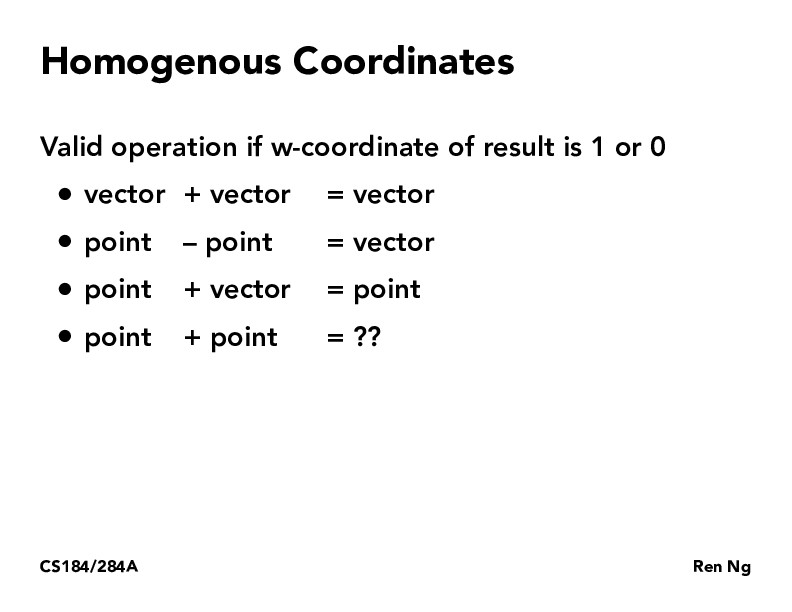Lecture 4: Transforms (24)bufudash

Why does point - point gives you a vector? And point + vector gives you a point? Isn't point a coordinate and how can it be added to a vector?jessicajyeh

A vector has magnitude and direction, so a point + vector can be thought of as: given a starting point, how far and in what direction do I go? The result is a point, since that is your ending position. Note that the equation for a ray (seen in lecture 10) is a point + a vector, where the point is the origin and the vector is the direction.

The difference between points can be thought of as, what would it take for me to get from point A to point B? A vector would do the trick. (You can also rearrange point + vector = point to get point - point = vector)

You must be enrolled in the course to comment